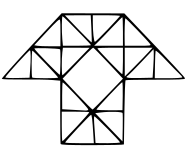0
67160

# SSC CGL Figure Counting Questions PDF:

Download SSC CGL Figure Counting questions with answers PDF based on previous papers very useful for SSC CGL exams. 25 Very important Figure Counting objective questions for SSC exams.

Question 1: How many triangles are there in this figure?

a) 10

b) 12

c) 14

d) 16

Question 2: How many triangles are  there in the given figure?

a) 12

b) 11

c) 10

d) 15

Question 3: How many triangles are there in this figure ?

a) 12

b) 16

c) 9

d) 8

Question 4: How many triangles are there in this geometric figure?

a) 12

b) 16

c) 18

d) 20

Question 5: How many triangles are there in the following figure?

a) 18

b) 13

c) 9

d) 5

Question 6: How many triangles are there in the question figure?

a) 6

b) 10

c) 12

d) 16

Question 7: How many triangles are there in the following figure?

a) 12

b) 16

c) 10

d) 20

Question 8: How many triangles are there in the given figure ?a) 29

b) 38

c) 40

d) 35

Question 9: How many triangles are there in the given figure ?

a) 32

b) 34

c) 37

d) 40

Question 10: How many triangles are there in the given figure ?

a) 24

b) 30

c) 28

d) 29

Question 11: How many triangles are there in the given figure.

a) 14

b) 15

c) 17

d) 18

Question 12: How many triangles are there in the given figure ?

a) 8

b) 10

c) 12

d) 14

Question 13: How many triangles are there in the given figure ?

a) 12

b) 13

c) 15

d) 18

Question 14: How many triangles are there in the given figure.

a) 4

b) 5

c) 6

d) 7

Question 15: How many triangles are there in the given figure?

a) 10

b) 11

c) 12

d) 13

Question 16: How many triangles are there in the given figure ?

a) 14

b) 15

c) 16

d) 19

Question 17: How many triangles are there in the given figure ?

a) 16

b) 20

c) 22

d) 24

Question 18: How many triangles are there in the given figure ?

a) 11

b) 12

c) 13

d) 15

Question 19: How many triangles are there in the given figure ?

a) 13

b) 14

c) 15

d) 16

Question 20: How many triangles are there in the given figure ?

a) 12

b) 13

c) 15

d) 18

Question 21: How many triangles are there in the given figure

a) 20

b) 23

c) 24

d) 26

Question 22: How many triangles are there in this given figure

a) 8

b) 9

c) 10

d) 12

Question 23: How many triangles are there in the given figure?

a) 18

b) 19

c) 20

d) 21

Question 24: How many triangles are there in the given figure ?

a) 28

b) 36

c) 40

d) 48

Question 25: How many triangles are there in the given figure ?

a) 38

b) 44

c) 46

d) 54

Small triangles = 8

Triangles formed by 2 triangles = 2

Triangles formed by 2 triangles and one trapezium = 2

Larger triangles = 2

Thus, total triangles = 8 + 2 + 2 + 2 = 14

=> Ans – (C)

Small triangles = (AEF, AEG, GDH, CFH), (IEK, IFL, JLH, JKG), (IOK, IOL, JOL, KOJ)

Big triangles = IKL, JKL, IJK, IJL

Thus, total triangles = 16

=> Ans – (B)

Smaller triangles = (ABC) , (ACD) , (ADE) , (FGJ) , (GHJ) , (HIJ)

Triangles made by combination of 2 triangles = (ABD) , (ACE) , (AFG) , (AGH) , (AHI) , (FHJ) , (GIJ)

Triangles made by combination of 3 triangles = (ABE) , (FIJ)

Triangles made by combination of 4 triangles = (AFH) , (AGI)

Triangles made by combination of 6 triangles = (AFI)

Thus, total triangles = 18

=> Ans – (C)

Small triangles = 6

Triangles made by combining 2 small triangles = 3

Triangles made by combining 3 small triangles = 6

Large triangles = 1

So, total = 6 + 3 + 6 + 1 = 16

=> Ans – (D)

Smaller triangles = 12

Triangles formed with 2 triangles = 4

Larger triangles (combining triangles at the centre) = 4

Thus, total triangles = 12 + 4 + 4 = 20

=> Ans – (D)

Smaller triangles = 6

Triangles formed by combination of 3 triangles = 2

Larger triangles (at the diagonals) = 4

Thus, total triangles = 6 + 2 + 4 = 12

=> Ans – (C)

Smaller triangles = 7

Triangles formed at diagonals of smaller square = 4

Triangles formed at diagonals of larger square = 4

Thus, total triangles = 7 + 4 + 4 = 15

=> Ans – (C)

Larger triangles = 3

Triangles formed with color combination (blue+red) , (red) and (red+yellow) = 3

Thus, total triangles = 3 + 3 = 6

=> Ans – (C)

Smaller triangles = 6

Triangles formed with combination of 2 triangles = 2

Triangles formed with combination of 3 triangles = 2

Larger triangle = 1

Thus, total triangles = 6 + 2 + 2 + 1 = 11

=> Ans – (B)

Smaller triangles = 6

Triangles at the diagonal of the square = 4

Triangles formed by combining 2 triangles (at the right) = 1

Triangles formed by combining 1 triangles and 1 quadrilateral (at the bottom) = 1

Triangles formed by combining 3 triangles = 1

Thus, total triangles = 6 + 4 + 1 + 1 + 1 = 13

=> Ans – (C)

Small triangles = 5

Triangles formed by combination of 2 triangles = 3

Triangles formed by combination of 3 triangles = 1

Thus, total triangles = 5 + 3 + 1 = 9

=> Ans – (B)